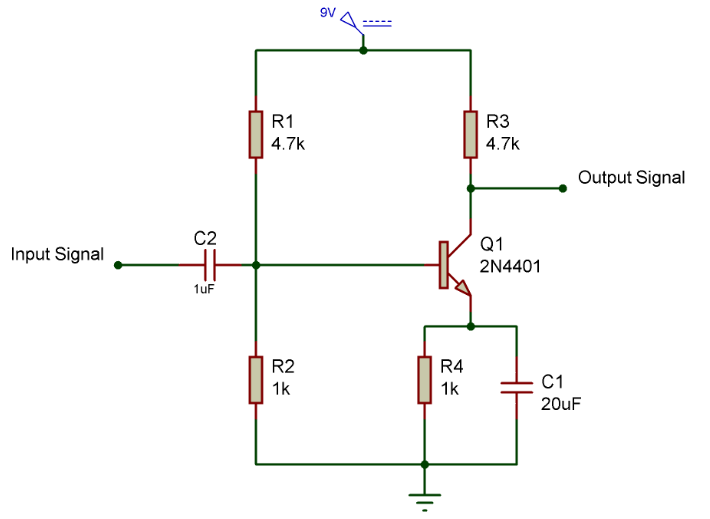# Amplifier Circuit using TransistorSingle Transistor Amplifier

Amplifiers play an important role in boosting up the signal to required level and widely employed in audio applications. Transistor amplifiers can be built with ease and also cost effective than other types. In order to built one all you need is Transistor, power supply, some Resistors and Capacitors. For complex amplifier circuits go for this 200W subwoofer Amplifier. Let’s move in to the working of amplifier circuit using transistor .

Design of an amplifier requires consideration of several parameters such as gain, current  and voltage obtained from it. The required parameters can be meet up by careful selection of components and simple math. This article will teach you through the steps involved in designing a amplifier using a single transistor.

## WORKING:

The above circuit uses a simple NPN Transistor Q1 2N4401 and powered by a 12v Supply. The resistor values R1,R2,R3,R4 and capacitor values C1,C2 plays major role in amplification of input signals. So fixing these values requires some simple math to meet our amplification requirements. First we need to fix the value of Collector resistor R3 based on the desired collector current and voltage.

Since we need the Transistor to amplify both the negative and positive component of a signal ,it is advised to give Collector voltage Vc equals to 1/2Vcc that is 4.5v. Thereby using ohms we obtain the R3 value

R3 = (Vcc – Vc) / Ic

R3 = (9v – 4.5v) / 1mA = 4500 Ohm

4.7K is the standard value of resistor hence 4.7K is employed in the circuit.

The Current flowing through the Collector terminal will be equal to the current flowing through the emitter terminal Ic = Ie. Resistance in the emitter is already built into the transistor and it is called as transresistance, which is calculated as.

R4 = 0.026V / Ie

R4  = 0.026V / 1mA = 26 Ohm

The gain of the amplifier circuit can be calculated using the formula

Gain = Rc / Re = R3 / R4

Since small resistance will create stability problem in circuit therefore we need to add our own resistance in the emitter terminal. We are going to use a 1K resistor in the emitter to provide stability in the amplifier circuit. But the increase in resistance will decrease the gain obtained and we are going to compromise it by adding a capacitor parallel to R4 forming a high pass filter.

Using high pass filter we can pass signals of assigned frequency. Here the RC values are selected in a way to permit signals of 300 Hz. This can be calculated by

f = 1/( 2 x pi x R x C )

Substituting the R, f values we can obtain the C value

300 Hz = 1/(2 x 3.14 x 1000 x C)

C = 0.000020F or 20uF

Now Components at the Collector and Emitter terminals are fixed still we need to set R1 and R2 values for biasing transistor.

Transistor will switch on only if we apply voltage push at its base and this is equal to 0.6v for silicon transistor. The voltage at base of a transistor is 0.6v higher than the voltage at emitter so

Vb = Ve + 0.6V

Vb= 1v + 0.6v = 1.6v

So we are about to employ a voltage divider to supply the voltage to the base of the transistor. The Resistor values R1 and R2 can be calculated by  using the formula

Vout = R2 x Vin / ( R1 + R2 )

Rearranging these equations,

R1/R2 = ( Vin – Vout ) / Vout

R1/R2 = ( 9v – 1.6v ) / 1.6v  = 4.6

Thus R1 needs to be 4.6 times greater than the R2 and approximating the values give R1 = 4.7K and R2 = 1K.

Capacitor C2 of 1uF was added to remove the  DC offset in the input signal and keep our circuit stable.

And that’s it our Single transistor Amplifier Circuit is ready. You can alter the values of RC by doing some math to meet your needs.

## JLCPCB - Only \$2 for PCB Prototype (Any Color)

Up to \$20 shipping discount on first order now: https://jlcpcb.com/quote1 Followers

Most reacted comment
2 Comment authorsRecent comment authors
Subscribe
Notify ofGuest
Sergey

Thank you!
It is interesting and instructive!
Please tell us how to calculate the resistor in the power circuit the gate mosfet.
Example: IR2104 – resistor (?) – gate MOSFETwpDiscuz